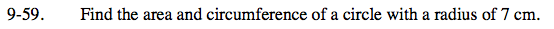Home > ACC7 > Chapter cc29 > Lesson cc29.2.1 > Problem9-59

9-59.

Find the area and circumference of a circle with a radius of 7 cm. Homework Help ✎Area of a circle = r2 π
Circumference = 2πr

Substitute 7 for r in each equation.

The area is approximately 153.9 cm2. What is the perimeter?